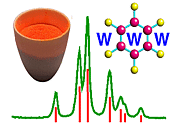Translational Symmetry II. Crystal Systems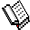Course Material Index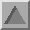Section Index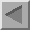Previous Page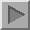Next Page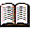The trigonal and hexagonal unit-cell information in the table below is reference material only.

Seven Crystal Systems

Most crystals usually have some type of rotational symmetry combined with the pure translational symmetry of the lattice. We now consider the constraints that rotational symmetry imposes upon lattices.

The figure below represents a two-dimensional section of the ab-plane of a lattice, red balls indicating equivalent lattice points. With no rotational symmetry constraints imposed upon the lattice, the angle γ may take any arbitrary value. Suppose a twofold rotation axis is combined with the lattice along the b-axis direction as shown below: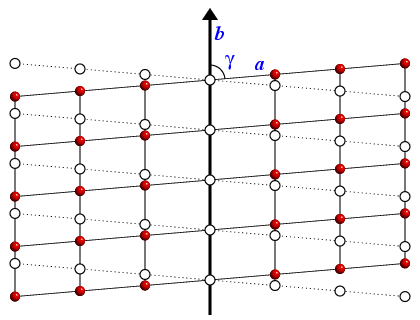Applying a twofold rotation to the lattices points generates the new lattice points indicated by open circles. The positions of the red balls and open circles will only be co-incident when the angle γ is constrained to be exactly 90°. Only when the angle is 90° can the lattice show twofold symmetry. So the obvious choice of unit cell is the one that constrains the angle γ to be 90°. A similar argument can be used to show that all unit-cell angles adjacent to a rotational axis are 90°.

Combining a lattice with the different orders of rotation symmetry leads to the seven possible crystal systems. The lattices of the seven crystal systems consist of an infinite array of identical points each with an identical environment. The point symmetry of this environment is known as the syngony of the lattice. The symbols of the syngonies will be explained later. The seven possible syngonies characterise the seven crystal systems:

Crystal System Characteristic
Symmetry
Syngony Unit-Cell Parameters Independent
Parameters
Triclinic 1×  1-fold -1 abc;  α ≠ β ≠ γ 6
Monoclinic 1×  2-fold 2/m abc;  α = γ = 90°; β ≠ 90° 4
Orthorhombic 3×  2-fold mmm abc;  α = β = γ = 90° 3
Tetragonal 1×  4-fold 4/mmm a = b ≠ c;  α = β = γ = 90° 2
Trigonal (see note) 1×  3-fold 6/mmm (P)
-3m   (R)
a = b ≠ c;  α = β = 90°;  γ = 120° 2
Hexagonal 1×  6-fold 6/mmm a = b ≠ c;  α = β = 90°;  γ = 120° 2
Cubic 4×  3-fold m-3m a = b = c;  α = β = γ = 90° 1

The characteristic symmetry indicates the minimal symmetry that is always present in each crystal system: n-fold can represent any type of symmetry axis of order n, e.g. the 2-fold in the monoclinic crystal system could be a twofold rotation axis, a two-one screw axis (see section on screw symmetry), a bar-two axis (see section on rotary-inversion symmetry), or a glide plane (see section on glide symmetry). Strictly speaking, "≠" in the above table should not be read as "does not equal" but "not constrained by symmetry to equal". Also the monoclinic cell is conventionally chosen to have the angle β greater than 90°.Note: In the trigonal system, the syngony of the lattice may have a higher symmetry than the characteristic symmetry of the crystal system depending on whether the lattice is primitive (P) or rhombohedrally centred (R).

The rhombohedral system (R) is a subgroup of the trigonal system in which either a triply-centred hexagonal cell may be used (as in the table) or, alternatively, a primitive rhombohedral cell may be used with a = b = c, α = β = γ ≠ 90°. The number of cell parameters is two irrespective of the choice of unit cell.Course Material IndexSection IndexPrevious PageNext Page
 © Copyright 1995-2006.  Birkbeck College, University of London. Author(s): Jeremy Karl Cockcroft Huub Driessen David Moss Andreas Wostrack